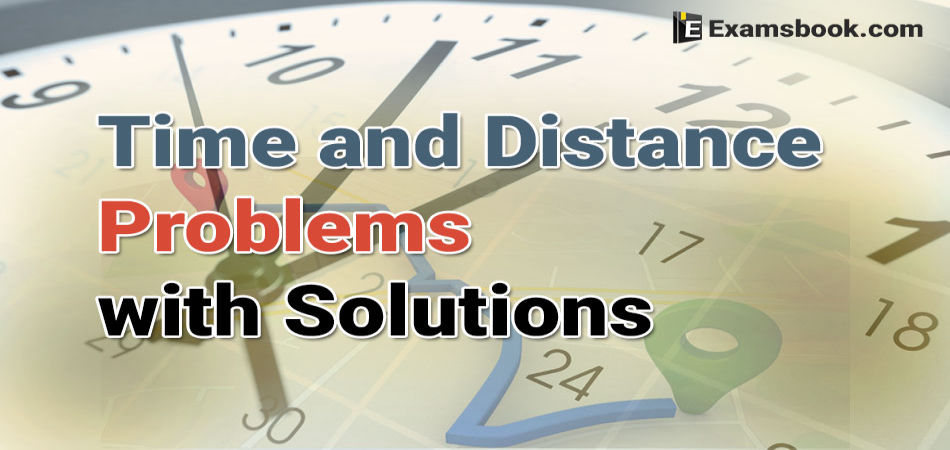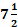• Save

# Time and Distance problems with solutions for bank exams

Vikram Singh11 months ago 5.0K ViewsUsually, students face difficulty while solving time and distance problems who are preparing for competitive exams because they don't know the correct way to solve time and distance problems with solutions. They got confused in calculating the time and distance but here in this blog, you can learn how to solve time and distance problems correctly.

These time and distance questions asked in competitive exams. So, you should learn to solve time and distance problems with solutions if you want to improve your performance in SSC and bank exams. You can practice time and distance questions with answers after learning how to solve properly.

## Time and Distance Problems with Solutions for Competitive Exams

Q.1. An athlete runs 200 metres race in 24 second. His speed is :-

Solution:

$$Speed = {200\over4}m/sec={25\over3}m/sec=\left({25\over3}×{18\over5} \right) km/hr=30km/hr$$

Q.2. A person crosses a 600m long street in 5 minutes. What is his speed in km per hour ?

Solution:

$$Speed = \left({600\over5×60} \right)m/sec=2 \ m/sec=\left(2×{18\over5} \right)=7.2 \ km/hr$$

Q.3.How long will a boy take to run round a square field of side 35 metres, if he runs at the rate of 9 km/hr ?

Solution:

$$speed = 9 km/hr =\left(9×{5\over18}\right)m/sec={5\over2}m/sec$$

$$Distance = (35×4)m=140m.$$

$$∴ Time \ taken= \left(140×{2\over5}\right)sec=56 \ sec$$

Q.4. One of the two buses complete a journey of 300 km inhours and the other a journey of 450 km in 9 hours. The ratio of their average speed is:

Solution:

$$Ratio\ of \ Speed =\left(300×{2\over15} \right):\left({450\over9} \right)=40:50=4:5$$

Q.5. The ratio between the speeds of two trains is 7 : 8. If the second train runs 400 kms in 4 hours, then the speed of the first train is:

Solution:

Let the speeds of two trains be 7x and 8x km/hr.

$$Then, 8x={400\over4}=100→x=\left({100\over8} \right)=12.5$$

If you have any doubt regarding time and distance problems with solutions for ssc and bank exams you can ask me in the comment section. Please visit on the next page.